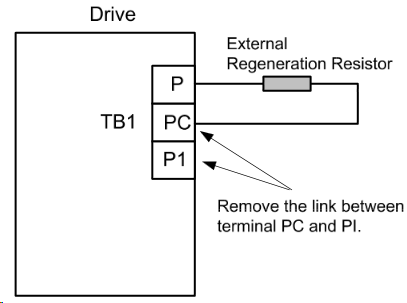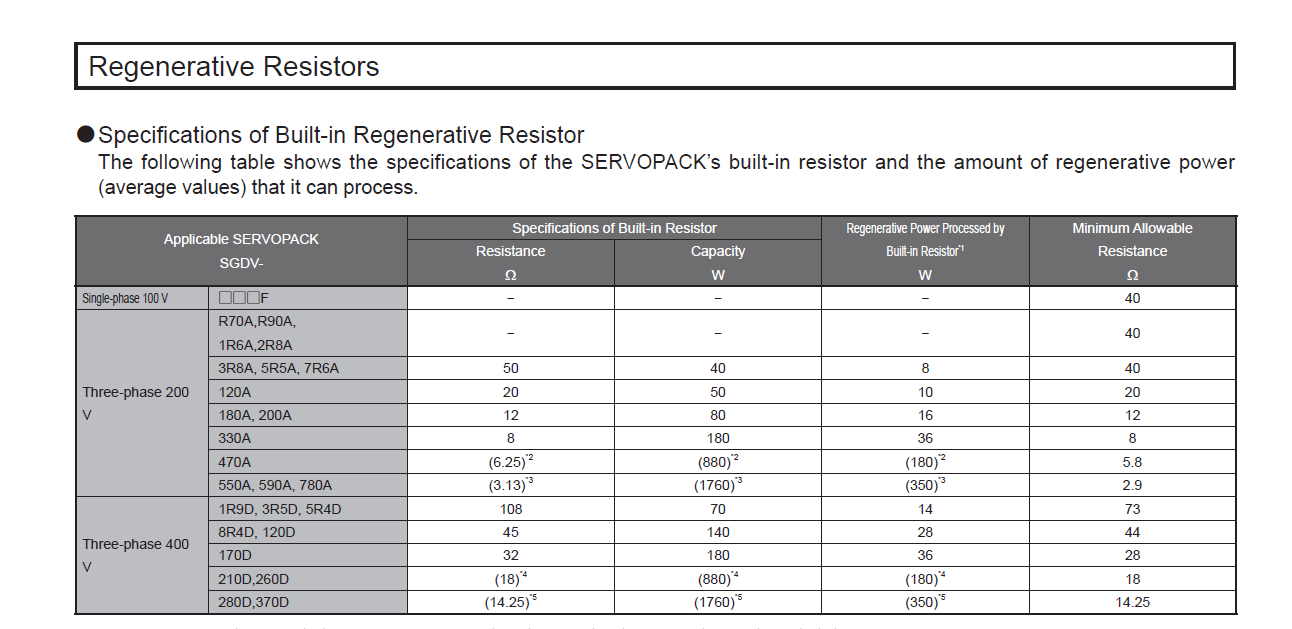# Regenerative Resistors

When installing Regeneration Resistors care must be taken as the resistor absorbs the regeneration power, and it is possible to generate high temperatures above 100°C.

Provide adequate cooling and use appropriate high temperature wires and ensure there is adequate space between regeneration resistor and other materials.  Install as far away from other components as possible to avoid electrical noise and heat damage.

#### For Teco:

• Most users will use the internal resistor; leave the jumper between PC-P1
• Persistent AL02 errors may require the addition of an external resistor to the servo drive
• For an external resistor, remove PC-P1 jumper and wire between P-PC
• Set Cn012 to the wattage of the external resistor (i.e. - Set Cn012 to 500 for a 500W resistor)
•• If the drive has an internal resistor, there will be a jumper between B2-B3 terminals
• Some servopack models do not have an internal resistor so an external regenerative resistor is required
• For an external resistor, remove B2-B3 if present and wire between B1-B2
• Set Pn600 with the Regenerative Resistor Capacity
• A.320 alarm is because the resistor wattage has not been programmed or not set correctly
• See pg. 3-41 and following.
• SETTING PN600:

The Yaskawa drives have a built-in resistor that is appropriate for most customers. In that case, Pn600 will be set to 0 and B2 will be jumpered to B3 on the drive.

If a larger external resistor is needed:

• The jumper between B2 and B3 will be removed and discarded
• The new external resistor will be wired to B1 and B2
• The units on Pn600 is 10, not 1.
• Pn600 will need to be set to 20% of the rated watts of the new resistor (for natural convection resistor).
• A common resistor is 50 ohms at 90 watts. So: 90 watts X 0.20 = 18 watts. Because the units on Pn600 is 10, instead of entering 18 you will want to enter 1.8, but that parameter needs to be a whole number. You will round to the nearest whole number and enter 2.
• Two resistors in series doubles both ohms and watts. Two resistors 5 ohms 6 watts in series: 10 ohms, 12 watts
two resistors in parallel cuts the ohms in half and doubles the watts. Two resistors 5 ohms 6 watts in parallel: 2.5 ohms, 12 watts

The Pn600 value will vary with the cooling method of external regenerative resistor:
• For natural convection cooling: Set the value to a maximum 20% of the actually installed regenerative
resistor capacity (W).
• For forced convection cooling: Set the value to a maximum 50% of the actually installed regenerative
resistor capacity (W).

The Minimum Allowable Resistance is the column that needs to be focused on.